# 生成模型的基本功能

• 密度估计
• 生成样本

## 密度估计

$p(y|x) = \frac{p(x|y)}{ \sum_{y}p(x,y)}$

## 生成样本

• 根据隐变量的先验分布$p(z,\theta)$进行采样，得到样本$z$
• 根据条件分布$p(x|z,\theta)$进行采样，得到样本$x$

# 变分自动编码器

## 从分布变换看VAE

VAE和GAN的目标都是：希望构建一个从隐变量$Z$生成目标数据$X$的模型，但是实现上有所不同。它们假设$Z$服从某些常见的数据分布（正态分布，均匀分布等），然后希望训练得到一个模型$X=g(Z)$，这个模型能够将原来的概率分布映射到训练集的概率分布，即，他们的目的都是进行分布之间的变换。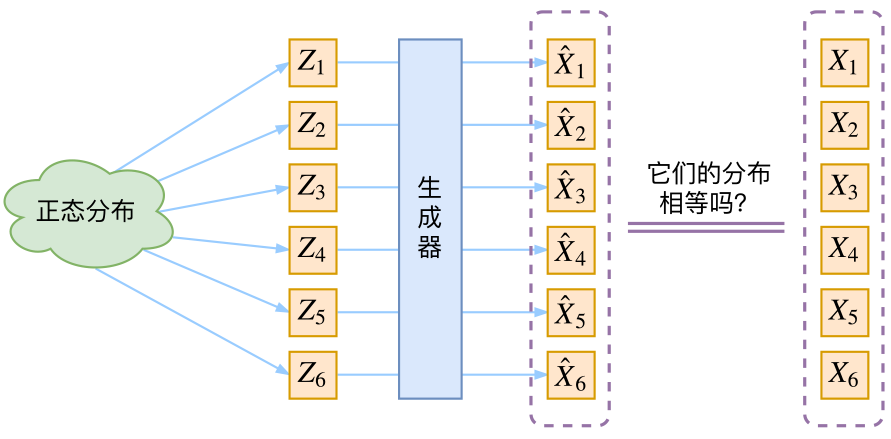## VAE的通常理解

$p(X)=\sum_{Z}p(X|Z)p(Z)$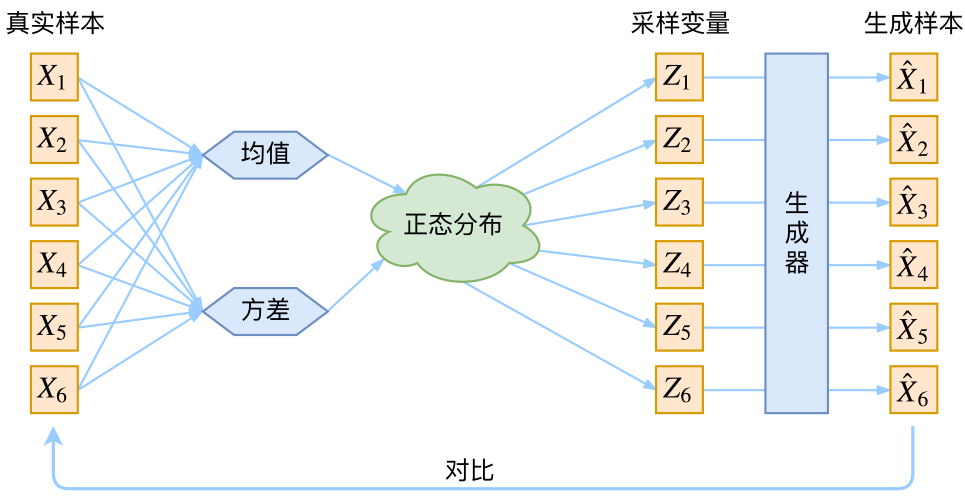## VAE应该是什么样的

In this case, we can let the
variational approximate posterior be a multivariate Gaussian with a diagonal covariance structure:
$\log q_{\phi}(\boldsymbol{z}|\boldsymbol{x}^{(i)}) = \log \mathcal{N}(\boldsymbol{z} ;\boldsymbol{\mu}^{(i)},\boldsymbol{\sigma}^{2(i)}\boldsymbol{I})\tag{9}$
（注：这里是直接摘录原论文，本文所用的符号跟原论文不尽一致，望读者不会混淆。）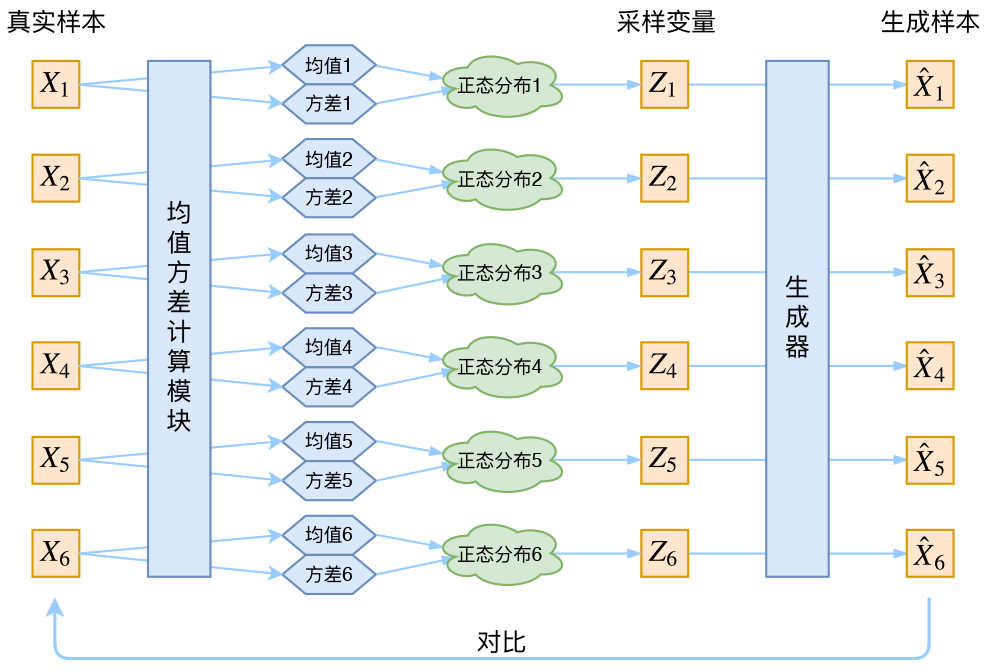## 分布标准化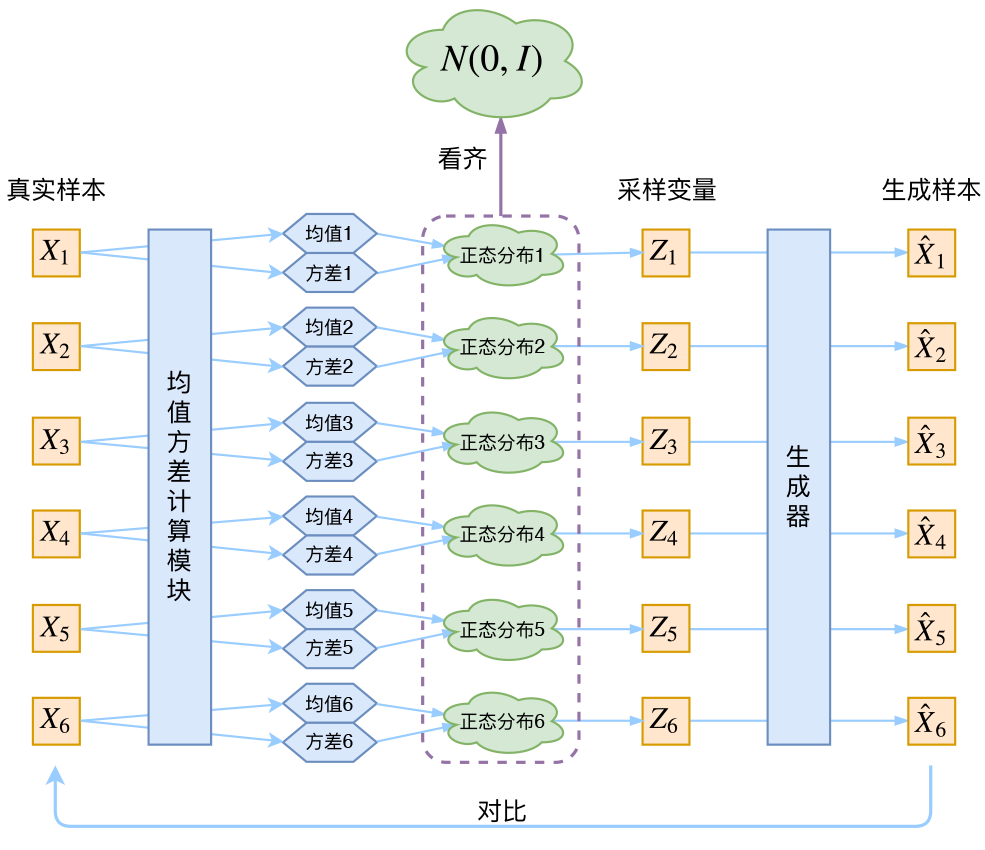$\mathcal{L}_{\mu,\sigma^2}=\frac{1}{2} \sum_{i=1}^d \Big(\mu_{(i)}^2 + \sigma_{(i)}^2 - \log \sigma_{(i)}^2 - 1\Big)\tag{4}$

## VAE的本质

VAE虽然也是AE（AutoEncoder）的一种，但他的做法是独特的，在VAE中，他的Encoder有两个，一个用来计算均值，一个用来计算方差。

VAE从让普通人望而生畏的变分和贝叶斯理论出发，最后落地到一个具体的模型中，虽然走了比较长的一段路，但最终的模型其实是很接地气的：他本质上就是在我们常规的自编码器的基础上，对encoder的结果加上了“高斯噪声”，使得结果decoder能够对噪声有鲁棒性，而那个额外的KL loss事实上就是相当于encoder的一个正则项，希望encoder出来的东西均有零均值。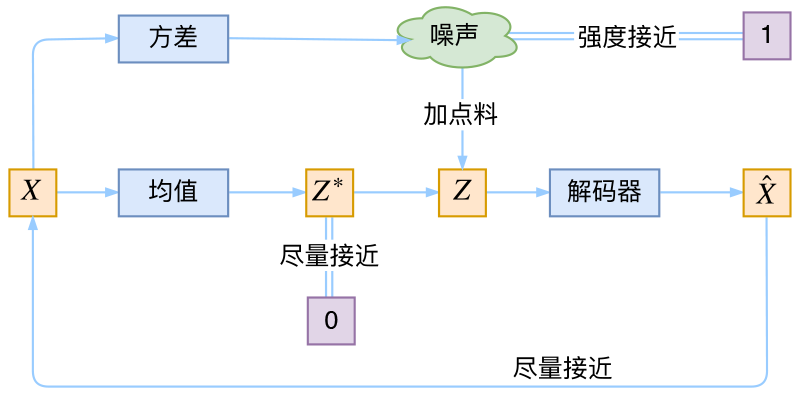## VAE中的变分

VAE叫做“变分自编码器”，他跟变分法有什么联系？在VAE的论文和相关解读中，好像也没看到变分法的存在呀？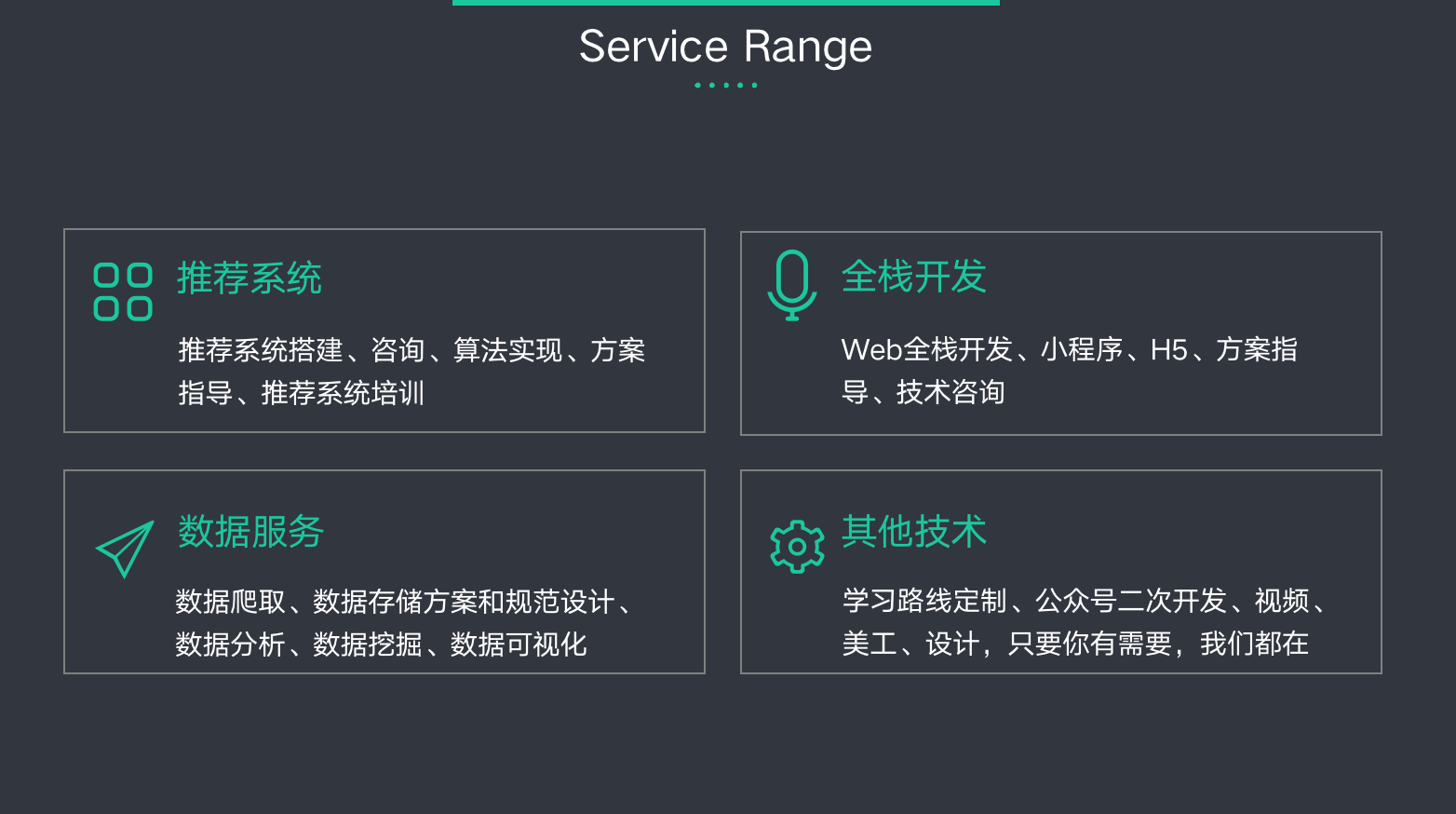©️2019 CSDN 皮肤主题: 大白 设计师: CSDN官方博客